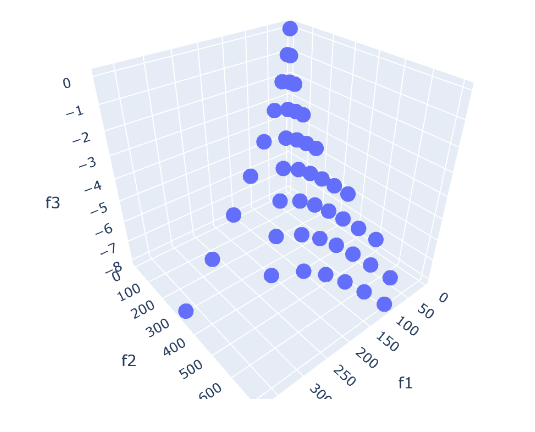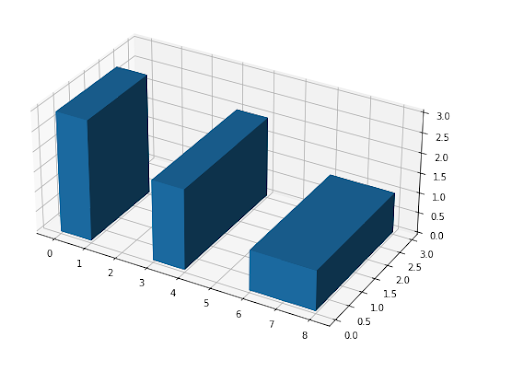## Thursday, October 21, 2021

### A multi-objective nonlinear integer programming problemPicture of the efficient points

In , a small multi-objective non-convex MINLP problem is stated:

Let's see how one could approach a problem like this.

## Saturday, October 16, 2021

### Stacking 3d boxes under rotationBoston custom house tower

Assume we have an infinite collection of 3d boxes of different sizes. As an example let's use :

----     30 PARAMETER boxes  available box sizes

size-x      size-y      size-z

box1           4           6           7
box2           1           2           3
box3           4           5           6
box4          10          12          32


We want to build a tower of boxes such that the base of the box on top is strictly smaller than the underlying box. The "strictly" part is essential. Otherwise, we can just repeat the same type of box ad infinitum. The interesting angle is: we can rotate the boxes. Without loss of generality, we can assume that for all boxes, after rotation, we have: the width ($$x$$) is less than the depth $$(y$$). (This may require a bit of thinking). With this, any box can be placed in just three different ways.3d rotations

The objective is to build a tower that is as tall as possible. In , this problem is solved using a Dynamic Programming algorithm. Here I want to see how this looks using a MIP model.

## Monday, October 11, 2021

### 2d knapsack problem

This post is about what sometimes is called a 2d knapsack problem. It can also be considered as a bin-packing problem. These are famously difficult problems, so let's have a look at a relatively small instance.

So, we have one 2d container of a given size. Furthermore, we have a collection of items: rectangles of a given size, with a value. The goal is to pack items in our container without overlapping each other such that the total value is maximized.

To make things concrete, let's have a look at a small data set (randomly generated):

----     18 PARAMETER data  problem data

width      height   available       value  value/size

k1             20.000       4.000       2.000     338.984       4.237
k2             12.000      17.000       6.000     849.246       4.163
k3             20.000      12.000       2.000     524.022       2.183
k4             16.000       7.000       9.000     263.303       2.351
k5              3.000       6.000       3.000     113.436       6.302
k6             13.000       5.000       3.000     551.072       8.478
k7              4.000       7.000       6.000      86.166       3.077
k8              6.000      18.000       8.000     755.094       6.992
k9             14.000       2.000       7.000     223.516       7.983
k10             9.000      11.000       5.000     369.560       3.733
container      30.000      20.000


## Tuesday, September 28, 2021

### Another Rook Tour on the Chessboard

In  the following problem is described:

1. The chessboard shown above has $$8\times 8 = 64$$ cells.
2. Place numbers 1 through 64 in the cells such that the next number is either directly left, right, below, or above the current number.
3. The dark cells must be occupied by prime numbers. Note there are 18 dark cells, and 18 primes in the sequence $$1,\dots,64$$. These prime numbers are: 2,3,5,7,11,13,17,19,23,29,31,37,41,43,47,53,59,61.

This problem looks very much like the Numbrix puzzle . We don't have the given cells that are provided in Numbrix, but instead, we have some cells that need to be covered by prime numbers.

The path formed by the consecutive values looks like how a rook moves on a chessboard. Which explains the title of this post (and of the original post). If we require a proper tour we need values 1 and 64 to be neighbors. Otherwise, if this is not required, I think a better term is: Hamiltonian path. I'll discuss both cases, starting with the Hamiltonian path problem.

### A covering problem: runners vs Ragnar relay legs

In  the following problem was posted.

1. We have a number of legs with their lengths (in miles). E.g.

legs = [3.3, 4.2, 5.2, 3, 2.7, 4,
5.3, 4.5, 3, 5.8, 3.3, 4.9,
3.1, 3.2, 4, 3.5, 4.9, 2.3,
3.2, 4.6, 4.5, 4, 5.3, 5.9,
2.8, 1.9, 2.1, 3, 2.5, 5.6,
1.3, 4.6, 1.5, 1.2, 4.1, 8.1]

2. We also have a number of runs performed by runners. Again, we have distances in miles:

runs = [3.2, 12.3, 5.2, 2.9, 2.9, 5.5]

A run can be allocated to different legs, as long a the sum of these legs is less than the length of the run.
3. We want to maximize the number of legs covered by the runs, but with a wrinkle: the legs should be consecutive: 1,2,3,... (we can't skip a leg).
4. A solution for this data set can look like:

----     60 PARAMETER report  results

run1        run2        run3        run4        run5        run6

leg1                       3.3
leg2                       4.2
leg3                                   5.2
leg4           3.0
leg5                                               2.7
leg6                       4.0
leg7                                                                       5.3
total          3.0        11.5         5.2         2.7                     5.3
runLen         3.2        12.3         5.2         2.9         2.9         5.5


It is noted that solutions are not necessarily unique. In this case, we could have swapped run 4 for run 5 to cover leg 5.

## Friday, September 17, 2021

### Reallocate people: a very small but interesting LPU-Haul Moving Van [link]

This is a strange little problem . We have individuals (of different categories) and regions with a given capacity. The initial set of assigned persons fits within the capacity of each region. Now a new batch of individuals arrives with their chosen zones. Unfortunately, the total demand (old + new individuals) does not fit anymore in all cases. This means we need to reallocate people. We can do this at a price (the costs are given). What is the optimal (least cost) new allocation?

## Friday, September 10, 2021

### Huber regression: different formulationsPeter Huber, Swiss statistician

Traditionally, regression, well-known and widely used, is based on a least-squares objective: we have quadratic costs. A disadvantage of this approach is that large deviations (say due to outliers) get a very large penalty. As a result, these outliers can affect the results dramatically.

One alternative regression method that tries to remedy this is L1- or LAD-regression (LAD=Least Absolute Deviation) . Here I want to discuss a method that is somewhere in the middle between L1 regression and least-squares: Huber or M-Regression .  Huber regression uses a loss function that is quadratic for small deviations and linear for large ones. It is defined in a piecewise fashion. The regression can look like the following non-linear optimization problem:

NLP Model
\begin{align} \min_{\color{darkred}\beta,\color{darkred}\varepsilon} &\sum_i \rho(\color{darkred}\varepsilon_i) \\ &\color{darkblue}y_i = \sum_j \color{darkblue}x_{i,j}\color{darkred}\beta_j +\color{darkred}\varepsilon_i \end{align} where $\rho(\color{darkred}\varepsilon_i) = \begin{cases}\color{darkred}\varepsilon^2_i & \text{if |\color{darkred}\varepsilon_i | \le \color{darkblue}k} \\ 2\color{darkblue}k |\color{darkred}\varepsilon_i |-\color{darkblue}k^2 & \text{otherwise}\end{cases}$

## Tuesday, August 31, 2021

### matrix operations via GAMS Embedded Python: multi-regional Input-Output tablesWassily Leontief in front of an IO table, credit:NYU

#### Inverting a dense matrix

GAMS allows running pieces of Python code as part of a GAMS model. Unfortunately, the interface is rather low-level and inefficient.  Usually "low-level" is associated with high-performance, but in the Python world, this is not the case. Here is an example.

## Sunday, August 15, 2021

### Stable Marriage ProblemAn inter-cast marriage ceremony in Lalitpur 

The Stable Marriage Problem is a fascinating problem. In this problem, we want to assign ("marry") men to women much like the assignment problem. (I am just following the conventions in the literature here.) There is a twist, however. We want to require that the matchings are stable: there does not exist a combination $$(m,w)$$ such that $$m$$ and $$w$$ both prefer each other above their current partner .

There are famous algorithms for this problem . But here, I want to look at it as an integer programming problem.

## Sunday, August 8, 2021

### A network model: Pyomo vs GAMS

Network models are an important class of optimization models, in itself but also as part of larger models. In  a nice presentation is given on how to set up a network model in the Python-based modeling tool Pyomo.

The simple shortest path problem is reproduced here: